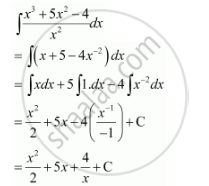Share

# Find the Following Integrals Int (X^3 + 5x^2 -4)/X^2 Dx - Mathematics

#### Question

Find the following integrals  int (x^3 + 5x^2   -4)/x^2 dx

#### SolutionIs there an error in this question or solution?
Find the Following Integrals Int (X^3 + 5x^2 -4)/X^2 Dx Concept: Integration as an Inverse Process of Differentiation.